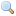# Module Complex

`module Complex: `sig` .. `end``
Complex numbers.

This module provides arithmetic operations on complex numbers. Complex numbers are represented by their real and imaginary parts (cartesian representation). Each part is represented by a double-precision floating-point number (type `float`).

``type t = {``
 `  ` `re :float;` `  ` `im :float;`
}
The type of complex numbers. `re` is the real part and `im` the imaginary part.
`val zero : `t``
The complex number `0`.
`val one : `t``
The complex number `1`.
`val i : `t``
The complex number `i`.
`val neg : `t -> t``
Unary negation.
`val conj : `t -> t``
Conjugate: given the complex `x + i.y`, returns `x - i.y`.
`val add : `t -> t -> t``
`val sub : `t -> t -> t``
Subtraction
`val mul : `t -> t -> t``
Multiplication
`val inv : `t -> t``
Multiplicative inverse (`1/z`).
`val div : `t -> t -> t``
Division
`val sqrt : `t -> t``
Square root. The result `x + i.y` is such that `x > 0` or `x = 0` and `y >= 0`. This function has a discontinuity along the negative real axis.
`val norm2 : `t -> float``
Norm squared: given `x + i.y`, returns `x^2 + y^2`.
`val norm : `t -> float``
Norm: given `x + i.y`, returns `sqrt(x^2 + y^2)`.
`val arg : `t -> float``
Argument. The argument of a complex number is the angle in the complex plane between the positive real axis and a line passing through zero and the number. This angle ranges from `-pi` to `pi`. This function has a discontinuity along the negative real axis.
`val polar : `float -> float -> t``
`polar norm arg` returns the complex having norm `norm` and argument `arg`.
`val exp : `t -> t``
Exponentiation. `exp z` returns `e` to the `z` power.
`val log : `t -> t``
Natural logarithm (in base `e`).
`val pow : `t -> t -> t``
Power function. `pow z1 z2` returns `z1` to the `z2` power.